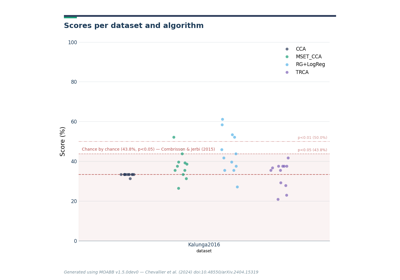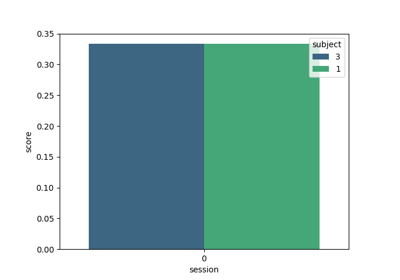class `moabb.paradigms.``SSVEP`(fmin=7, fmax=45, **kwargs)[source][source]

Single bandpass filter SSVEP

SSVEP paradigm with only one bandpass filter (default 7 to 45 Hz) Metric is ‘roc-auc’ if 2 classes and ‘accuracy’ if more

Parameters
• fmin (float (default 7)) – cutoff frequency (Hz) for the high pass filter

• fmax (float (default 45)) – cutoff frequency (Hz) for the low pass filter

• events (list of str | None (default None)) – List of stimulation frequencies. If None, use all stimulus found in the dataset.

• n_classes (int or None (default None)) – Number of classes each dataset must have. All dataset classes if None

• tmin (float (default 0.0)) – Start time (in second) of the epoch, relative to the dataset specific task interval e.g. tmin = 1 would mean the epoch will start 1 second after the begining of the task as defined by the dataset.

• tmax (float | None, (default None)) – End time (in second) of the epoch, relative to the begining of the dataset specific task interval. tmax = 5 would mean the epoch will end 5 second after the begining of the task as defined in the dataset. If None, use the dataset value.

• baseline (None | tuple of length 2) – The time interval to consider as “baseline” when applying baseline correction. If None, do not apply baseline correction. If a tuple (a, b), the interval is between a and b (in seconds), including the endpoints. Correction is applied by computing the mean of the baseline period and subtracting it from the data (see mne.Epochs)

• channels (list of str | None (default None)) – List of channel to select. If None, use all EEG channels available in the dataset.

• resample (float | None (default None)) – If not None, resample the eeg data with the sampling rate provided.

Attributes
`datasets`

Property that define the list of compatible datasets

`scoring`

Property that defines scoring metric (e.g.

Methods

 `get_data`(dataset[, subjects, return_epochs]) Return the data for a list of subject. `is_valid`(dataset) Verify the dataset is compatible with the paradigm. `prepare_process`(dataset) Prepare processing of raw files `process_raw`(raw, dataset[, return_epochs]) Process one raw data file.
 used_events

## Examples using `moabb.paradigms.SSVEP`¶